# [Maya C++ API] paint weights with MPxDeformer# Polar decomposition of a 3x3 matrixLeaving [C++ code ] to decompose a 3x3 matrix into rotation and scale/shear with polar decomposition (this is done through a 3x3 SVD and 3x3 Eigen decomposition).

Keywords:

• Polar decomposition of a 3x3 matrix
• 3x3 matrix SVD (Singular Value decomposition)
• Eigen decomposition (extract eigenvalues and eigenvectors) of a 3x3 matrix M (assumes symmetric matrix)

# [Maya C++ API] Get selected vertices of a mesh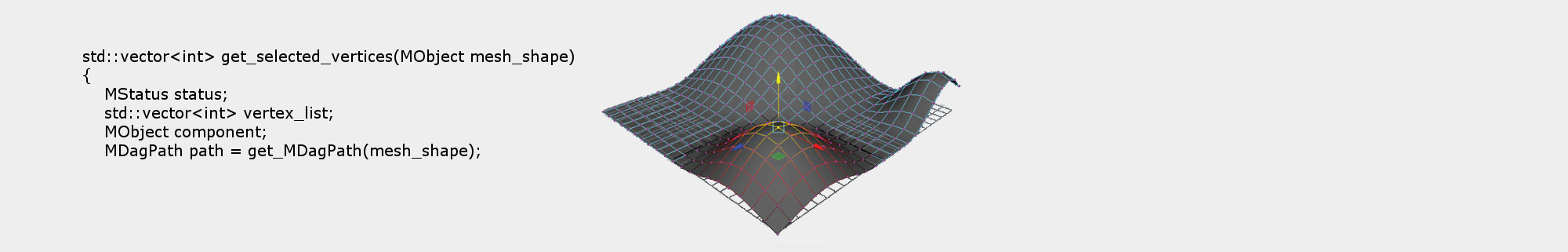# [Maya C++ API] Set skinning weight attributes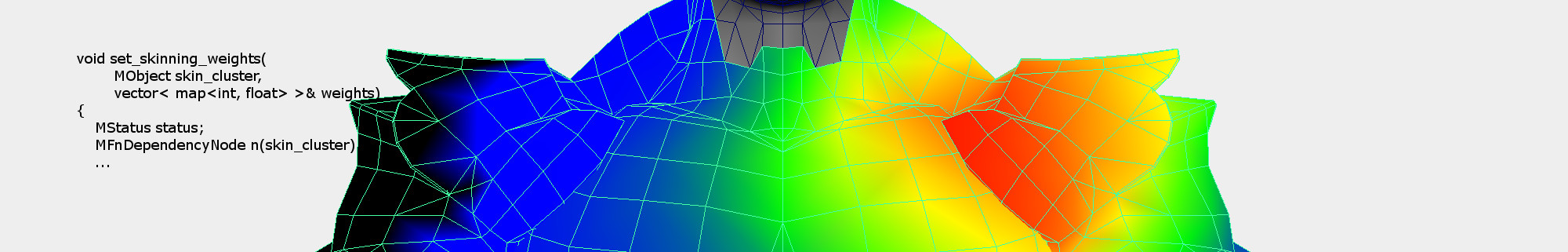Some C++ Maya API code to set skin weights (multi attributes) of a skin cluster node.

# Bulge free Dual Quaternion Skinning (Trick)C++ code snippet to correct the Dual Quaternion Skinning bulge artifact. Left: standard DQS, right bulge correction, (automatic skin weight with Maya "smooth bind").

# Laplacian smoothing (C++ code to smooth a mesh)Dropping a procedure to compute the Laplacian smoothing of a 3D mesh (with cotangent weights).

# C++ code for cotangent weights over a triangular mesh$$w_{ij} = \cot(\alpha_{ij}) + \cot(\beta_{ij})$$

# Implicit skinning routines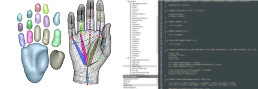Here you will find our Implicit skinning standalone application under the license GNU GPL3.

Disclaimer
The standalone application is written in Qt/C++ and CUDA, therefore it only works on NVIDIA GPUs but should run on Windows or Linux. This code is a research project developed on three Linux machines with similar configurations and "tested" by very few people: expect difficulties to get it to work. The documentation is also very thin. That being said I will be happy to answer questions in case of troubles.

# Voro++.0.4.5 with cmake for easy compilation under windows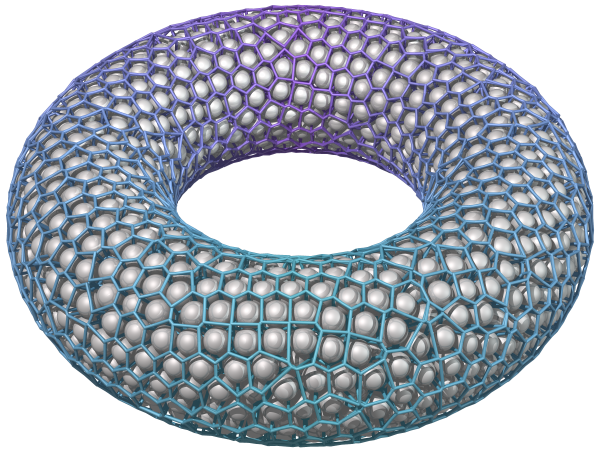Dropping my code of the [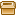cmake version of voro++0.4.5 ]. It helped me to compile the voro++ library under windows with cmake.

# Singular value decomposition of a 2x2 matrix (C++ code)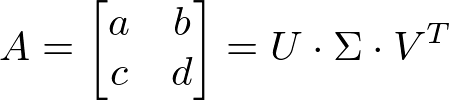Dropping some [code here] to do a singular value decomposition (SVD) of a 2 by 2 real matrix.

# Source code for poisson disk sampling of a triangle mesh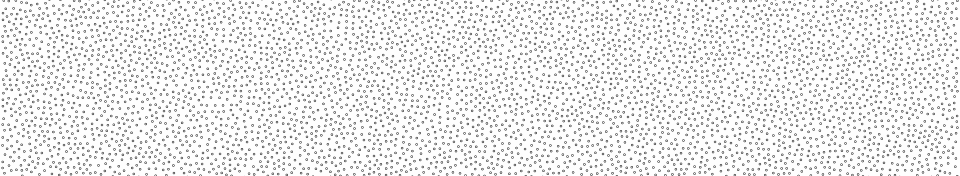Here you candownload the C++ code] to do a Poisson disk sampling of a triangular mesh. The code is a simple wrapper for the vcglib library. It takes in input a std::vector of triangles and vertex position/normals and outputs std::vector of samples positions/normals computed with the Poisson disk sampling.

EDIT: Victor Martins pointed out that the above code doesn't compile on MAC and kindly provided an [update] bundled with a newer version of vcglib.

N.B: if you are looking for a way to do Poisson disk sampling fast/in real-time take a look at this siggraph paper and matlab code. There is also a C++ implementation here.

# 3D viewer for Hermite Radial Basis Function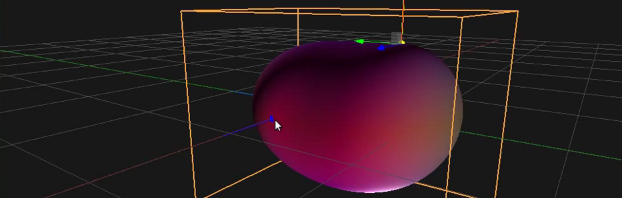This is a simple demonstrator of the HRBF technique presented and explained here. You can visualize and edit the implicit surface generated with HRBF.

# C++ code for spline curves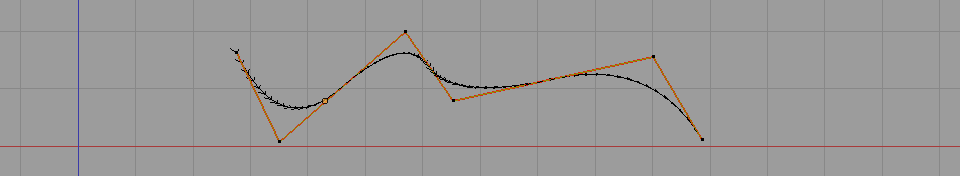Dropping on github C++ code to compute spline curves of arbitrary dimensions 3D curves or 2D splines anything is possible. The class can be instantiated with any point type (1d (float), 2d, 3d etc.) as long as the appropriate operator overload are implemented. This class use the efficient blossom algorithm to compute a position on the curve.

# Trackball C++ code without quaternions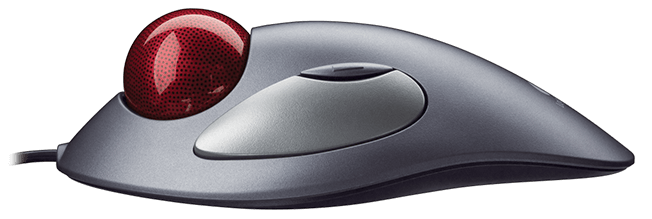I'm leaving some code to handle a simple trackball. The code actually wasn't done by me, but I can't remember where I took it! Originally it was C code which I've wrapped up in a C++ class with way more comments and an example of how to use it. [C++ trackball code ].

# C++ code to emulate openGL old direct mode drawing

glBegin(GL_TRIANGLES);
glVertex3f(1.0f, 0.0f, 0.0f);
glVertex3f(0.0f, 1.0f, 0.0f);
glVertex3f(0.0f, 0.0f, 1.0f);
glEnd();


[compact version ] | [modular version ]

Remember the old days when you were able to simply draw a few primitives with GL_POINTS, GL_LINES or GL_QUADS within a pair of good old begin() end(). Well I'm providing a C++ class which will enable you to do this again under OpenGL 3.1 or higher.

# C code for 4x4 matrix inversion

Just leaving some code here to invert either column or row major 4x4 matrices.

• 1